# Insert a vertical bar with integration limits into the equation

Word
For some standards, it is necessary to add to the equation the vertical bar with integration limits. Microsoft Word doesn't propose the simple way how to add it.

For example, the Newton-Leibnitz formula: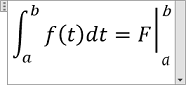Sure, you can add the vertical bar and superscript and subscript, but the formula look is not perfect: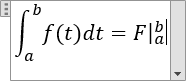To create a good-looking vertical bar with integration limits, do the following:

1.   Add the formula in your document as usual (see how to add different types of equations).

2.   In the place, where it is needed, add the vertical line by typing:

• \vert,
• \vbar or
• use the symbol Vertical line in the keyboard.

3.   Add superscript and subscript as a matrix: under Equation Tools, on the Design tab, in the Structures group, click the Matrix button. In the Matrix list choose 3x1 Empty Matrix: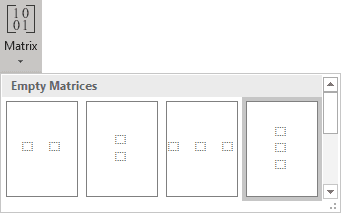• In the top box, type the symbol b,
• In the middle box, type the symbol space,
• In the bottom box, type the symbol a.

4.   Format the vertical line:

4.1.   Select the vertical line: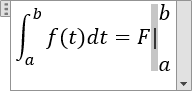4.2.   Under Equation Tools, on the Design tab, in the Conversions group, click the button Text to highlight it: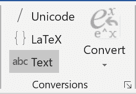4.3.   Change the font and its size as you prefer.

See also Adjusting spacing and alignment in an equation to customize the look of integration limits after the vertical line.

See also this tip in French: Insérer une barre verticale avec des limites d'intégration dans l'équation.